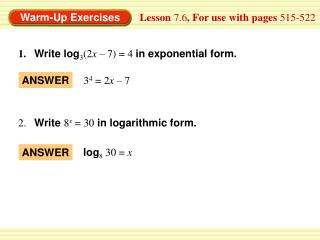DownloadDownload PresentationLesson 7.6 , For use with pages 515-522

# Lesson 7.6 , For use with pages 515-522

Télécharger la présentation## Lesson 7.6 , For use with pages 515-522

- - - - - - - - - - - - - - - - - - - - - - - - - - - E N D - - - - - - - - - - - - - - - - - - - - - - - - - - -
##### Presentation Transcript

1. ANSWER 34 = 2x – 7 log8 30 = x ANSWER Lesson 7.6, For use with pages 515-522 1.Write log3(2x – 7) = 4 in exponential form. 2. Write 8x= 30 in logarithmic form.

2. ANSWER 3 2 1 ANSWER 125 Lesson 7.6, For use with pages 515-522 Solve the equation. 3. 100x= 1000 4.log5x = –3

3. – 5, 12 ANSWER Lesson 7.6, For use with pages 515-522 Evaluate the logarithm. 5.x2 – 7x – 60 = 0